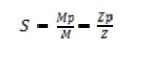Home | | Structural Analysis II | Important Questions and Answers: Plastic Analysis Of Structures

# Important Questions and Answers: Plastic Analysis Of Structures

Civil - Structural Analysis - Plastic Analysis of Structures

P LASTIC ANALYSIS OF STRUCTURES

1. What is a plastichinge?

When a section attains full plastic moment Mp, it acts as hinge which is called a plastic hinge. It is defined as they ieldedzone due to bending at which large rotation s can occur with a constant value of plastic moment Mp.

2. What is a mechanism?

When an-degree indeterminate structure develops n plastic hinges, it becomes determinate and the formation of an additional hinge will reduce the structure to a mechanism. Once a structure becomes a mechanism, it will collapse.

3.  What is difference between plastic hinge and mechanical hinge?

Plastic hinges modify the behavior of structures in the same way as mechanical hinges. The only difference is that plastic hinges permit rotation with a constant resisting moment equal to the plastic moment Mp. At mechanical hinges, theresisting moment is equal to zero.

The load that causes the(n +1) th hinge to form a mechanism is called collapse load where n is the degree of statically indeterminacy. Once the structure becomes a mechanism

5. List out the assumptions m adefor plastic analysis.

The assumptions for plastic analysis are:

Plane transverse sections remain plane and normal to the longitudinal axis before and after bending.

Effect of shear is neglected.

The material is homogeneous and isotropic both in the elastic and plastic state.

Modulus of elasticity has the same value both in tension and compression.

There is no resultant axial force in the beam.

The cross-section of the beam is symmetrical about an axis through its centroid and parallel to the plane of bending.

6. Define shape factor.

Shape factor(S) is defined as the ratio of plastic moment of the section to the yield moment of the section.Where Mp = Plastic moment

M=Yield moment

Zp = Plastic section modulus

Z= Elastic section modulus

7. List out the shape factors for the following sections.

(a)Rectangular section S =1.5 (b)Triangular section S =2 .346 (c)Circular section S =1.697 (d)Diamond section S =2

8. Mention the section having maximum shape factor.

The section having maximum shape factor is a triangular section, S =2.345.

Load factor is defined as the ratio of collapse load to working load and is given by10.            State upper bound theory.

Upper bound theory states that of all the assumed mechanisms the exact collapse mechanism is that which requires a minimum load.

11.            State lower bound theory .

Lower bound theory states that the collapse load is determined by assuming suitable moment distribution diagram. The moment distribution diagram is drawn in such away that the conditions of equilibrium are satisfied.

12.            What are the different types of mechanisms?

The different types of mechanisms are:

Beam mechanism

Column mechanism

Panel orsway mechanism

Cable mechanism

Combined or composite mechanism

13Depending on the support and load conditions indicate the possible locations of plastic hinges.

14.            Mention the types of frames.

Frames are broadly of two types:

(a)Symmetric frames

(b)Un-symmetric frames

15.            What are symmetric frames and how they analyzed?

Symmetric frames are frames having the same support conditions, lengths and loading conditions on the columns and beams of the frame. Symmetric frames can be analyzed by:

(a)Beam mechanism

(b)Column mechanism

16.            What are unsymmetrical frames and how are they analyzed?

Un-symmetric frames have different support conditions, lengths and loading conditions on its columns and beams. These frames can be analyzed by:

(a)Beam mechanism

(b)Column mechanism

(c)Panel orswaymechanism (d)Combined mechanism

17.            Define plastic modulus of a section Zp.

The plastic modulus of a section is the first moment of the area above and below the equal are a axis. It is the resisting modulus of a fully plasticized section.

Zp =A/2 (Z1+Z2)

18.            How is the shape factor of a hollow circular section related to the shape factor of a ordinary circular section?

The shape factor of a hollow circular section =A factor K x shape factor of ordinary circular section.

SF of hollow circular section =SF of circular section x{(1- c3)/(1- c4)}

19.            Give the governing equation for bending.

The governing equation for bending is given by M/I=s/y

Where M=Bending moment I=Moment of inertia

s=Stress

y=c.g. distance

20.            Give the theorems for determining the collapse load.

The two theorems for the determination of collapse load are:

(a)Static Method [Lower bound Theorem]

(b)Kinematic Method [Upper bound Theorem]

Study Material, Lecturing Notes, Assignment, Reference, Wiki description explanation, brief detail
Civil : Structural Analysis : Plastic Analysis Of Structures : Important Questions and Answers: Plastic Analysis Of Structures |